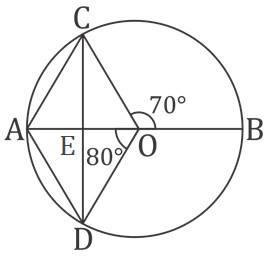Question of The Day27-07-2020

In the given figure, what will be the measure of the ∠ECB – ∠ BAC? (Given: O is the centre of the circle)Correct Answer : a ) 15o

Explanation :In the given figure,

We know that the angle subtended on the circumference of the circle is half the angle subtended at the centre of the circle by the same arc.

So,

∠BAC=$${1 \over 2}$$∠BOC=$${1 \over 2}$$*70=35o………1

Similarly,

⇒∠DCA=$${1 \over 2}$$∠DOA=$${1 \over 2}$$*80=40o

We know that, angle subtended by the semicircle/diameter on the circumference is 90 degree

So, ∠BCA = 90o

Now, ∠ ECB = ∠ BCA – ∠ DCA = 90o – 40o = 50o ……….  (2)

Therefore, from equation 1 and 2

∠ECB – ∠ BAC = 50o – 35o = 15o

Hence, (a) is the correct answer.

Such type of question is asked in various government exams like SSC CGL, SSC MTS, SSC CPO, SSC CHSL, RRB JE, RRB NTPC, RRB GROUP D, RRB OFFICER SCALE-I, IBPS PO, IBPS SO, RRB Office Assistant, IBPS Clerk, RBI Assistant, IBPS RRB OFFICER SCALE 2&3, UPSC CDS, UPSC NDA etc.

Read Daily Current Affairs, Banking Awareness, Hindi Current Affairs, Word of the Day, and attempt free mock tests at PendulumEdu and boost your preparation for the actual exam.0